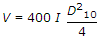# Civil Engineering - Water Resources Engineering - Discussion

### Discussion :: Water Resources Engineering - Section 1 (Q.No.39)

39.

With the usual meanings ofletters, the equationis used for determining the velocity of ground water flow in metres per day. It is known as

 [A]. Meinzer's formula [B]. Slichter's formula [C]. Darcy's formula [D]. Hazen's formula.

Explanation:

No answer description available for this question.

 Ramesh said: (Jan 28, 2017) It should be "mu" not 4".

 Sweetyakhi said: (Mar 20, 2017) Slichter's formula(c) = 2.303 (A/t)log10 * (s1/s2).

 Hagos said: (Aug 27, 2018) I think Darcy formula, v=ki. Manning's formula, v=1/n*r^2/3*s^1/2.